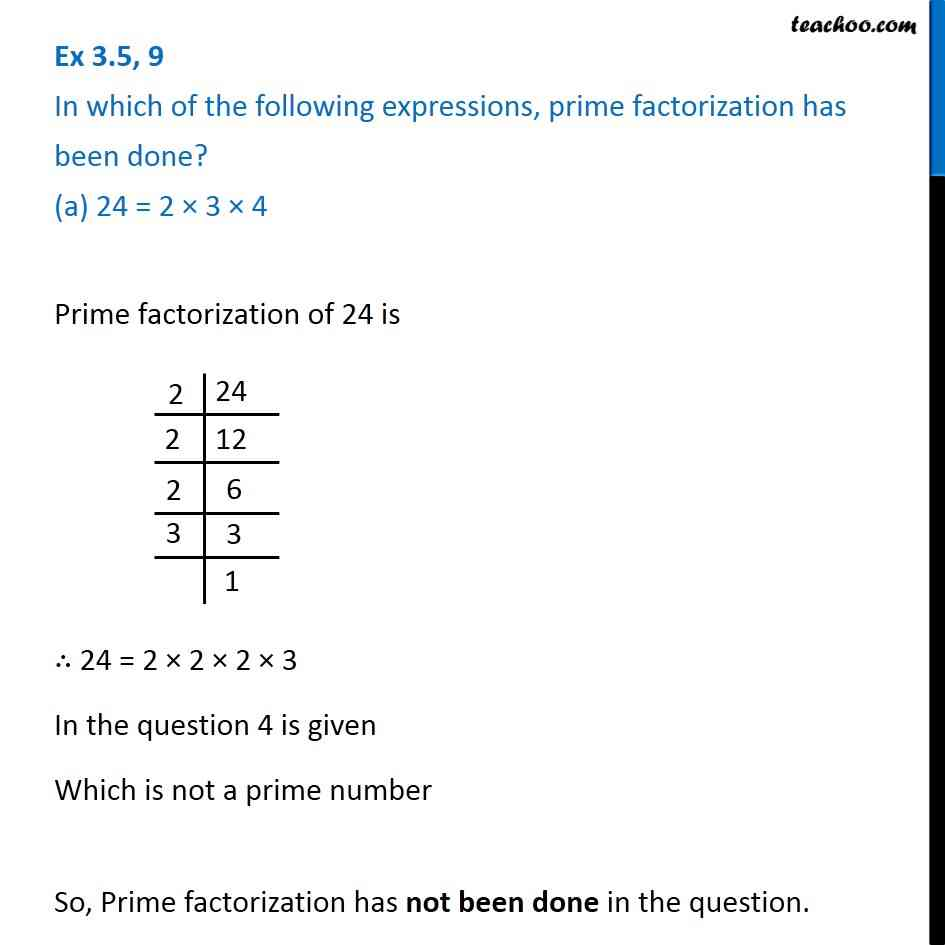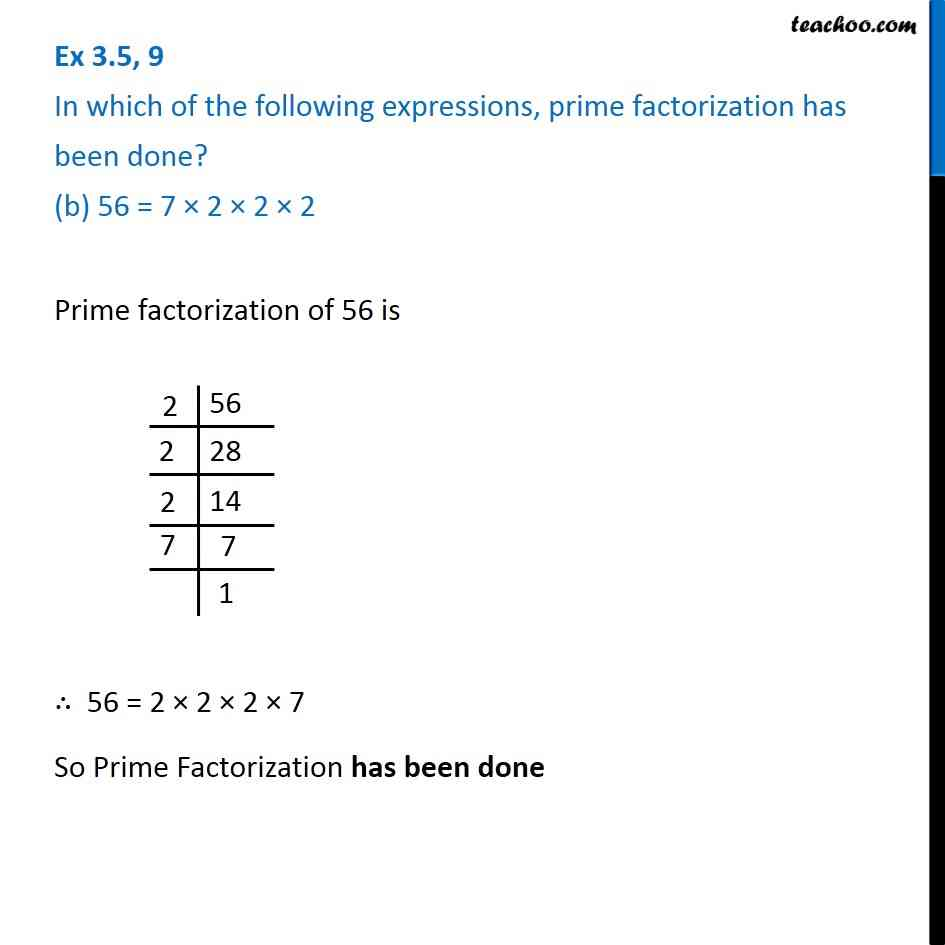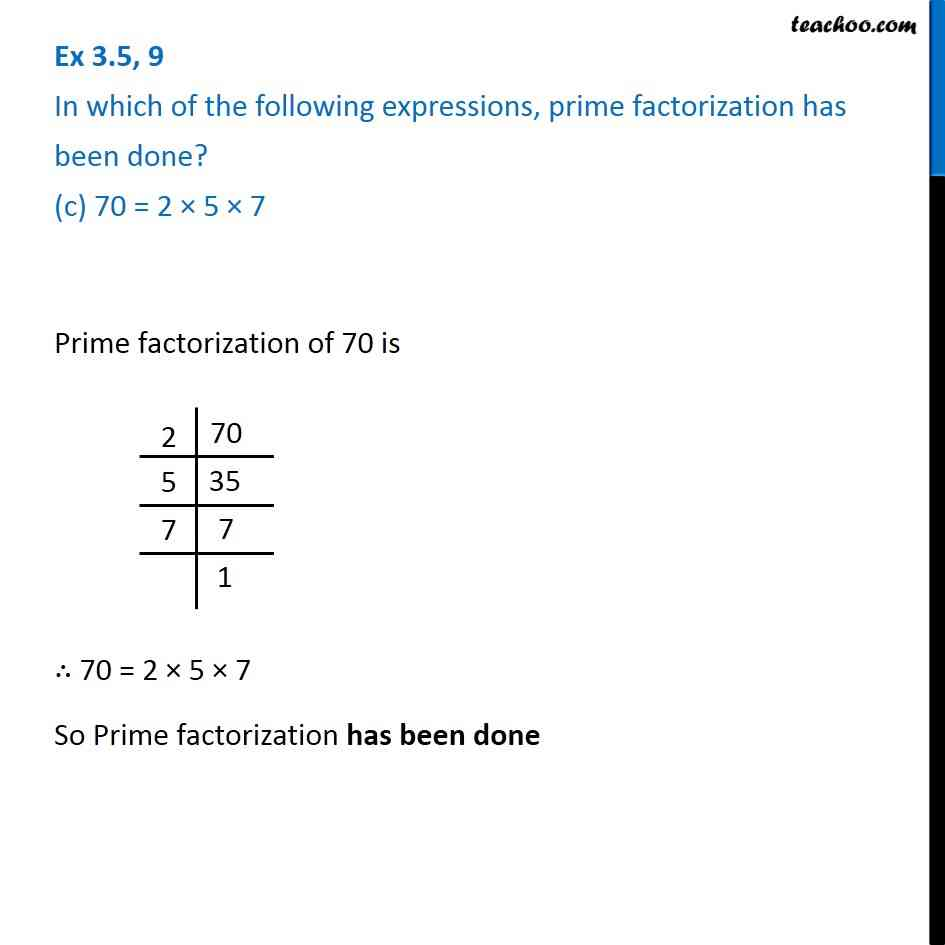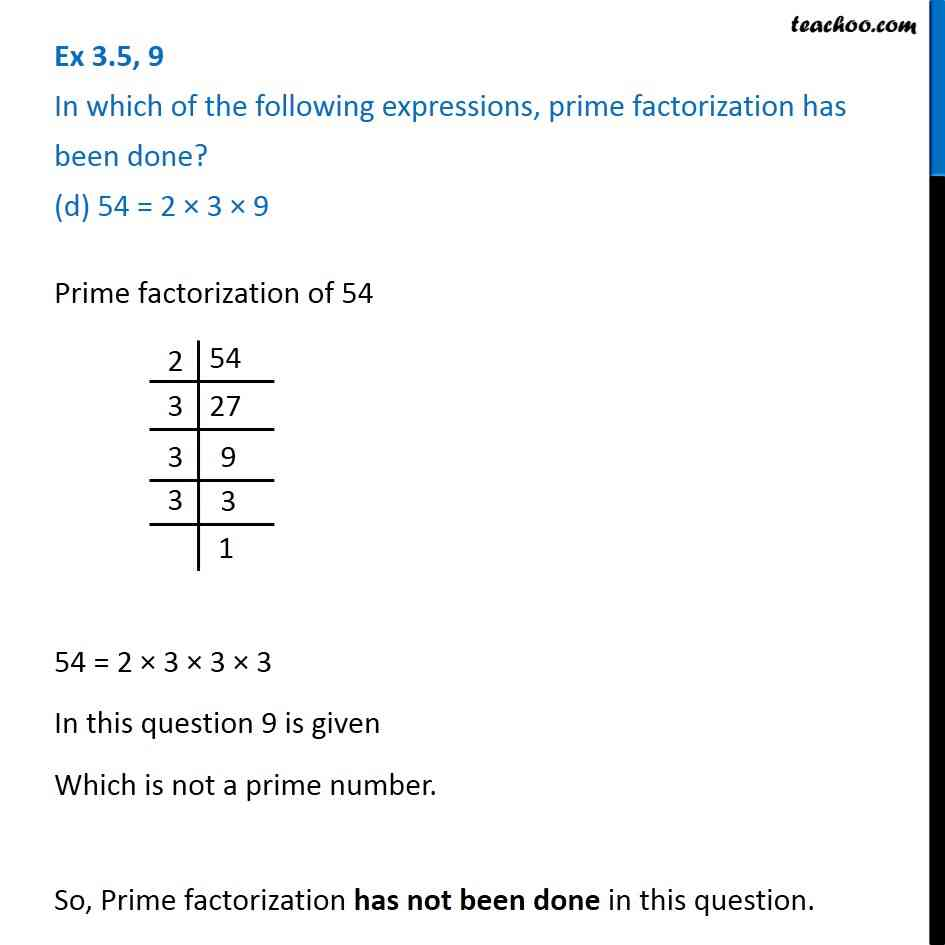1. Chapter 3 Class 6 Playing with Numbers
2. Concept wise
3. Prime Factorisation

Transcript

Ex 3.5, 9 In which of the following expressions, prime factorization has been done? (a) 24 = 2 × 3 × 4Prime factorization of 24 is ∴ 24 = 2 × 2 × 2 × 3 In the question 4 is given Which is not a prime number So, Prime factorization has not been done in the question. Ex 3.5, 9 In which of the following expressions, prime factorization has been done? (b) 56 = 7 × 2 × 2 × 2Prime factorization of 56 is ∴ 56 = 2 × 2 × 2 × 7 So Prime Factorization has been done Ex 3.5, 9 In which of the following expressions, prime factorization has been done? (c) 70 = 2 × 5 × 7 Prime factorization of 70 is ∴ 70 = 2 × 5 × 7 So Prime factorization has been done Ex 3.5, 9 In which of the following expressions, prime factorization has been done? (d) 54 = 2 × 3 × 9Prime factorization of 54 54 = 2 × 3 × 3 × 3 In this question 9 is given Which is not a prime number. So, Prime factorization has not been done in this question.

Prime Factorisation